Smartick is a fun way to learn math!Aug16

# Review Factoring with Examples

Hello again! Do you know what factoring is? Do you know what it is used for? In this post we will answer these questions.

### How to Factor

The factorization of a number is performed by writing the number as the multiplication of all its prime factors.

Example:

12 = 2 x 2 x 3

You can also express it with powers:

12 = 2 2 x 3

Now that we know how to factor, let’s see what it is useful for and how we can use it.

### To Calculate the Least Common Multiple (LCM)

The LCM of a set of numbers is calculated by factoring all the numbers. Once factored, common multiples with the greatest exponent and multiples that are not common are chosen. They are multiplied and the result is the LCM of those numbers.
Example:

LCM (12, 20)

We factor the numbers:

12 = 2 2 x 3

20 = 2 2 x 5

Now we choose the common factors (22) and non-common factors (3 and 5)
Multiply the factors:

2 2 x 3 x 5 = 60

Therefore, the LCM (12, 20) = 60

### To Calculate the Greatest Common Factor (GCF)

The GCF is calculated by factoring all numbers. Once factored, the common factors raised to lowest exponent are chosen. After that, the factors are multiplied.
Example:

GCF (30, 40)

We factor the numbers:

30 = 2 x 3 x 5

40 = 2 3 x 5

Now we choose the highest common factors raised to the lowest exponent (2 and 5)
Multiply the factors:

2 x 5 = 10
Therefore, the GCF (30, 40) = 10

### To Simplify Fractions

Fractions are simplified by dividing numerator and denominator by the same number, until they have no common factors. Using factoring in this case is very simple: we factor the numerator and denominator, then cancel out the common factors, and finally multiply the remaining factors.
Example: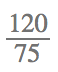First factor the numerator and the denominator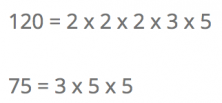Now cancel out the factors that are both in the numerator and denominator.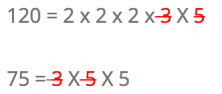The factors that remain are the factors that we must multiply.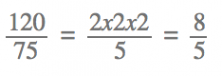And that’s the simplified fraction!

### To Perform Multiplication

There are some multiplications that may be easier if factoring is done first since factors can be conveniently grouped.
Example:

25 x 12

We factor the numbers:

25 = 5 x 5

12 = 2 x 2 x 3

Therefore, 25 x 12 = 5 x 5 x 2 x 2 x 3

We take a 2 and a 5 on one hand, and the remaining factors on the other.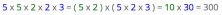This way we can perform the multiplication in a much simpler way.

In general, factoring can be used to simplify numerical calculations. Can you think of any other way to use factoring? Share it with us!

And you already know: to continue learning about factoring and all subjects of mathematics, sign up at Smartick and become a math genius .